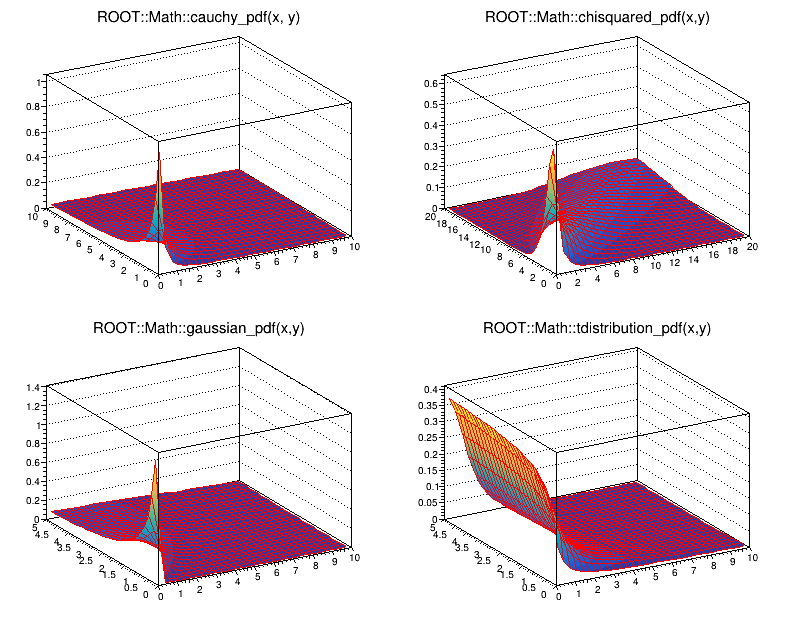# mathcoreStatFunc¶

Example macro showing some major probability density functions in ROOT. The macro shows four of them with respect to their two variables. In order to run the macro type:

root  .x mathcoreStatFunc.C


Original tutorial by Andras Zsenei.

Author: Alberto Ferro
This notebook tutorial was automatically generated with ROOTBOOK-izer from the macro found in the ROOT repository on Wednesday, August 17, 2022 at 09:34 AM.

In :
import ROOT

f1a = ROOT.TF2("f1a","ROOT::Math::cauchy_pdf(x, y)",0,10,0,10)
f2a = ROOT.TF2("f2a","ROOT::Math::chisquared_pdf(x,y)",0,20, 0,20)
f3a = ROOT.TF2("f3a","ROOT::Math::gaussian_pdf(x,y)",0,10,0,5)
f4a = ROOT.TF2("f4a","ROOT::Math::tdistribution_pdf(x,y)",0,10,0,5)

c1 = ROOT.TCanvas("c1","c1",800,650)
c1.Divide(2,2)
c1.cd(1)
f1a.SetLineWidth(1)
f1a.Draw("surf1")
c1.cd(2)
f2a.SetLineWidth(1)
f2a.Draw("surf1")
c1.cd(3)
f3a.SetLineWidth(1)
f3a.Draw("surf1")
c1.cd(4)
f4a.SetLineWidth(1)
f4a.Draw("surf1")

Welcome to JupyROOT 6.27/01


Draw all canvases

In :
from ROOT import gROOT
gROOT.GetListOfCanvases().Draw()